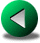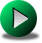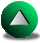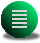Previous: The Time Discretization
Next: Finite Element Models
Up: Presentation of the Numerical

Subsections

# Finite Difference Models

## Introduction

The order of a finite-difference (FD) model is given by a Taylor series expansion of the numerical formulation. For instance, the first derivative of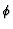given by a three point (equally spaced) formula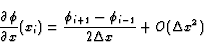(2.17)

ensures a second order accuracy. This means that if resolution is doubled, accuracy increases by a factor of 4. Higher order formulations are possible [Dietrich et al. , 1993], but most FD ocean models use second order schemes. In more than one dimensional problems, the best accuracy is obtained by using Cartesian-like grids (this includes curvilinear grids). And if there is any stretching of the grid, a change of less than 5% to 10% in size is usually recommended between two neighboring computational cells.

When finite difference models make use of Cartesian-like grids, a complex coastline is represented by a series of artificial steps''. More precisely, where the orientation of the boundary does not correspond to that of the grid, discretization of the boundary introduces a series of artificial steps'' along the coast (see Fig.2.1). Curvilinear models exist that tend to follow the coastline, but they usually fail as soon as the complexity of the coastline is too large (too many capes and bays). A dramatic example is the description of straits when only few points are available (Fig.2.2). In that situation, the strait width must take values in a set of discrete numbers at the price of misrepresenting the width and therefore the exchange of water masses. We are concerned with the issue of coastline representation in FD models and, particularly, we want to investigate the accuracy of FD models in presence of step-like lateral walls. These steps can be viewed as singularities (tips of land) around which the oceanic currents flow. A question therefore arises concerning the influence of resolution versus the influence of steps; the smaller the grid cell, the larger the number of steps along a coastline. It is then not clear whether the solution becomes more accurate (due to higher precision in the interior) or less accurate (due to an increased number of singularities or steps along the boundaries). If the model solution is less accurate with increasing resolution in presence of steps means that the model formulation becomes inconsistent in presence of steps. This may occur because FD models are made to be consistent in open or closed rectangular domains but are not necessarily in the more general case of irregular domains. In particular, we raise the problem of the computation of the advective and viscous terms in presence of steps. On the other hand, consistency should apply to the linear inviscid SW models.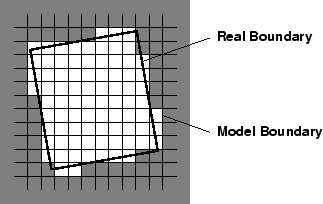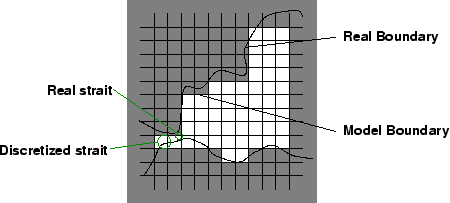The same problem arises in the vertical discretization of the topography in three-dimensional FD models of the ocean. In models of Bryan-Cox type [Bryan, 1969] based on the primitive equations, the vertical axis is discretized at various constant depths. They are called leveled or z-coordinate models. In these models, the topography follows a step-like representation and therefore they are prone to problems similar to the ones mentioned above. For instance, the equivalent difficulty in z-coordinate models to the description of straits is the description of sills. The depth of sills or other important topographical features has to be taken from a set of discrete depths. It was early realized that this step-like representation had detrimental effects on the overall circulation. For instance, z-coordinate models have meridional circulations which are known to be sensitive to the details of how the bottom boundary is represented. The issue is that they do not accurately advect denser waters along slopes and overestimate diapycnal mixing [Gerdes, 1993,Roberts et al. , 1996,Roberts and Wood, 1997]. Different strategies were proposed to circumvent the problem. The first strategy was to change the vertical coordinate, z, to a following terrain coordinate,[Phillips, 1957,Blumberg and Mellor, 1983]. But-coordinate models encounter other known limitations, such as pressure gradient errors and artificial diapycnal mixing. A second strategy is to use a layered (or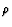-coordinate) model [Bleck, 1978,Bleck and Boudra, 1981]. Roberts et al. roberts96 compared the behavior of the simulated North Atlantic in a z-model and in an isopycnal model (-model). In particular, they noted that the z-model has more trouble in representing a realistic outflow from the Greenland basin (GIN). Roberts and Wood roberts97 extended the study by systematically studying the effect of modifying the topography of the sill at the outflow of GIN and noted the high sensitivity of the model. The same observation was made by Winton win97 in a more idealized geometry of the North Atlantic. Winton et al. win98 finally demonstrated that it is a resolution problem. When the resolution was high enough to resolve the bottom boundary layer and to resolve the slope, the flow is realistic enough. However, the required resolution is unrealistic even for modern z-models; therefore, they recommended the use of explicit bottom boundary layer models or the use of isopycnal models (although those ones have also their own limitations, namely related to the isopycnal layers intersecting the topography or the surface.) From a different perspective, Hirst and McDougall hirst96 noted that, in coarse resolution z-models, the Gent and McWilliams gent90 turbulence scheme remarkably enhances the conservation of water properties along topographical slopes. Another approach was proposed by Adcroft et al. adcro97. They showed interesting use of shaved'' cells in z-models. The topography is then piecewise linear, instead of being piecewise constant as in usual z-models.

All these difficulties in representing flows along sloping topography should warn us of possible problems for the horizontal circulation in the presence of step-like coastlines. Using a shallow water model, Schwab and Beletsky schwab98 found that a Kelvin wave moving along a coastline is sensitive to the presence of steps. The steps have mainly a retardation effect on the wave, the effect diminishing with higher resolution. These results are reproduced in Figure 2.3 using the C-grid model of Section 2.2.2. Four grids in total were used: two grids with no rotation of the basin showing no step along the boundaries at 10 and 5 km resolution and two grids with a 30orotation of the basin relative to the discretization axes showing steps along the walls at also 10 and 5 km resolution. That higher resolution decreases the retardation effect is consistent with the idea that Kelvin waves should not be sensitive to coastline details, at scales small compared to the Rossby radius of deformation. In Figure 2.3, for the highest resolution runs (5 km), the retardation effect is still noticeable but it is much weaker compared to the runs at 10 km resolution. Since the radius of deformation is 31 km in these runs, these results imply that we should resolve the Rossby radius with about ten points for a second order formulation. This retardation effect was also noted in circular lakes by Beletsky et al. belet97 for different kinds of staggering of the grid and vertical representations. One consequence for modelling the ocean is that the fast modes of an ocean basin (the Kelvin modes) will be misrepresented, especially if the model resolution is coarse. Therefore, transient responses of the ocean, such as the El-Niño Kelvin wave along the Western America may be retarded, which may have consequences on the period of occurrences of El-Niño events according to the delayed oscillator theory [Schopf and Suarez, 1988]. For instance, in the study of Soraes et al. soares99, there are only two points to represent the Rossby radius of deformation at 20o North. This means that their results are questionable concerning the flux and the timing of Kelvin waves leaving the equator and going poleward.

Figure 2.3: Elevation field for the Kelvin retardation problem in presence of steps along the walls at two different resolutions.represents the rotation angle of the grid relative to the discretization axes. a, 10 km,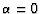; b, 10 km,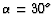; c, 5 km,; d, 5 km,. The dashed line is the -0.01 m contour, the solid lines are contours from 0.1 to 1.0 m with an increment of 0.1 m.a bc d

## The Three Staggerings Used

To ensure stability in primitive variable or shallow water models, the variables are usually staggered in space, in the sense that the discrete location of the different variables may differ. Several standard staggering techniques are used in ocean modelling: the non-staggered A-grid [Dietrich et al. , 1993], the B-grid [Bryan, 1969,Cox, 1984] or the C-grid [Bleck and Boudra, 1981,Blumberg and Mellor, 1983], as illustrated in Fig. 2.4. The A-grid leads to spurious modes of oscillation, fed by non-linear interactions and round-off errors. These spurious modes are ultimately unstable, but the A-grid can be stabilized if higher order formulations are used. The B-grid has better dispersion errors at coarse resolution for propagating planetary or Rossby waves than C-grid, and does worse for pure gravity waves [Batteen and Han, 1981].

Figure 2.4: The three major horizontal staggerings for the primitive equations. Left the A-grid, center the B-grid, right the C-grid. Velocities components are located by the arrows, the pressure or elevation point is located by a grey disk.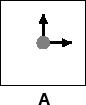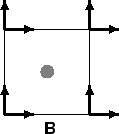FD models can be formulated to conserve energy and/or enstrophy [Arakawa, 1966,Sadourny, 1975,Abramopoulos, 1988,Arakawa and Hsu, 1990,Hólm, 1996]. For instance, it is relatively easy to formulate an A-grid energy conserving model, from the point of view of the finite volume (FV) method. But conserving the energy exactly only retards the occurrence of spurious numerical noise (this model is detailed in Appendix A). If the model was also enstrophy conserving (which, according to Abramopoulos, 1988, is achievable but very expensive), the occurrence of spurious numerical noise would be even more difficult and hence, the model would be stabilized.

### The C-grid Formulation

The C-grid derived models, such as the popular POM family of models developed from Blumberg and Mellor blum83, tend to be used preferentially for high-resolution regional studies. The C-grid FD model used in this thesis is the one formulated by Sadourny sadourny75. This model is enstrophy conserving. The nonlinear terms are split into a gradient term and a rotational term. To simplify the following discussion, we leave the time derivative being continuous. Using standart notation, the discretized shallow water equations are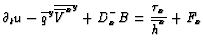(2.18)(2.19)(2.20)

The discretized potential vorticity is given by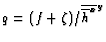whereis the relative vorticity. The discretized mass fluxes are given by,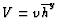, the discretized Bernouilli function is given by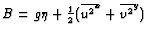and Fx and Fy are the viscous forces. The off-centered differencing operators in the x direction are defined by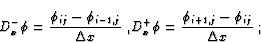and the averaging operator defined by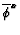is a double point average =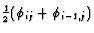. Similar definitions apply along the y direction. (2.18), (2.19) and (2.20) ensure a second order accuracy to the computation of the velocity and elevation fields. The kinematic boundary condition is no normal flow and the dynamic boundary condition is free-slip, unless otherwise specified. The C-grid model, in which the non-linear terms have been split into a rotational part and a gradient part, requires that vorticity be specified at boundary points. We set the relative vorticity to zero along the model boundary, which is consistent with the free-slip boundary condition along straight walls.

### The B-grid

The B-grid is employed in the popular MOM family of ocean models. The MOM model is a z-model and was developed from Bryan and Cox bryan67 and Bryan bryan69 and following investigators. The B-staggering suits more naturally the no-slip boundary condition, since the velocity points are located at the corners of the computational cell. Unlike the C-grid, there are no ambiguities in the way the dynamical boundary condition is imposed at tips of the continents. The B-grid is also well known for having a better dispersion relationship for Rossby waves at very coarse resolution than does the C-grid [Batteen and Han, 1981]. This makes this staggering technique more suitable for coarsely-resolved global climate studies. However, we are interested in how this configuration behaves in the presence of steps along the walls. From Cox cox79, it appears that the B-grid model under the no-slip boundary condition, just as the C-grid [Adcroft and Marshall, 1998], is not very sensitive to the presence of lateral steps, therefore, we prefer to focus on the behavior of the B-grid model with a free-slip boundary condition.

On the B-grid, the discretized shallow water equations become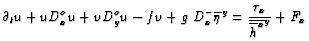(2.21)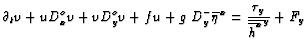(2.22)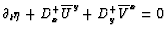(2.23)

where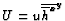,. The differencing operator Dox (and Doy in the similar way) are defined by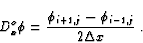Eq. (2.21) and (2.23) ensure a second order accuracy to the numerical solution. The difficulty when applying the free-slip boundary condition to a B-grid model is that it requires a prognostic equation for the velocity component tangential to the wall (in the more general situation of a irregular geometry, the B-grid would require equations for velocity nodes at tips of land-cells). Therefore we use,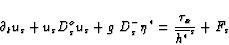(2.24)

where s represents the tangential direction, and, the elevation point along the wall at half a point from the considered velocity node.

### The A-grid

The argument behind using an A-grid configuration is that the C-grid presents the disadvantage of separate locations for u and v-components of the velocity. This means that, at coarse resolution, the truncation errors in the computation of the Coriolis terms can be fairly large. According to Adcroft et al. adcro98b, these errors trigger numerical noise when the Rossby radius is not well resolved. From a programming point of view, having all the variables located at the same points makes everything easier (physical parameterizations, conservative FV formulation, graphic output, ...). The A-grid arrangement of the variables is known to be an unstable second order formulation. Nonetheless, it is possible to run an A-grid model if all the terms are accurate at fourth order. A high-order method is cost effective in terms of accuracy [Sanderson, 1998], as long as the physical processes are resolved and the spectrum of the resolved fields is steep enough. Dietrich et al. die93, hereafter D93, developed such a model. The model is three-dimensional and uses a no-slip boundary condition. We modify the model to represent the shallow water equations, keeping the fourth order formulation for all the terms (except the diffusion), and we incorporate the free-slip boundary condition. All the equations are prognostic and integrated explicitly in time using a 4th order Runge-Kutta scheme. On an A-grid and using the same notation, the shallow water equations lead to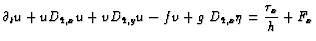(2.25)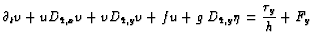(2.26)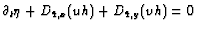(2.27)

The differencing operators, D4,x and D4,y, are fourth order operators. Equations 2.25-2.27 ensure a fourth order accuracy to the numerical solution, except for the viscous terms Fx and Fy, which remain second order. The difficulty with the A-grid at fourth order is to retain the fourth order right to the wall. This is possible only if off-centered differentiation formulae and interpolation are used. If this is not done, the model tends to be unstable with free-slip boundary conditions (as demonstrated in the next chapter.)Previous: The Time Discretization
Next: Finite Element Models
Up: Presentation of the Numerical

Frederic Dupont
2001-09-11